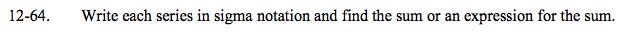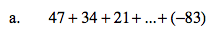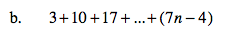### Home > A2C > Chapter 12 > Lesson 12.1.4 > Problem12-64

12-64.
1. Write each series in sigma notation and find the sum or an expression for the sum. Homework Help ✎

1. 47 + 34 + 21 + … + (−83)

2. 3+10 + 17 + … + (7n − 4)What is the expression for the sequence?

How many terms are there in the series?

$\sum_{k = 1}^{11}(60-13k)=-198$